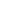HOMEAPPLICATIONWater treatment
Chemical Compatibility
This chart is intended only as a Guide. Users should verify chemical compatibility based upon experimentation with specific filter under actual use conditions:
chemical compatibility is affected by many variables, including temperature, concentration and length of exposure.
- Chemical compatibility data :This data is presented as a customer service. Accuracy can not be guaranteed.
- Variables in customer use such as concentrations, purity, temperature, pressure, time and various chemical combinations prevent complete accuracy.
Data Interpretation Chemical Compatibility Observations are Divided into Three Categories as Follows :
R - Recommended : No significant changes observed in performance, physical properties, dimensions or visible indication of chemical attack of the cartridge filter.
LR - Limited Recommended : Moderate changes in physical properties or dimensions of the cartridge filter were observed.
Filter may be suitable for short-term exposure at low pressure and ambient temperatures.
NR - Not Recommended : The cartridge filter is basically unstable. In most cases, extensive shrinking or swelling occurs. Filter may gradually weaken or partially dissolve after extended exposure Temperatures.
Filter media
 Chemicals PP PES PTFE Acids PP Acetic acid, glacial R R R Acetic acid, 90% R R R Acetic acid, 30% R R R Acetic acid, 10% R R R Hydrochloric acid, conc R - R Hydrochloric acid, 6N R R R Nitric acid, conc. R - R Nitric acid, 6N R R R Sulfuric acid, conc. R R Sulfuric acid, 6N R R Phosphoric R R Chromic acid, conc. R Hydrofluoric acid, 6N R R R Alcohols Amyl alcohol R R R Benzyl alcohol, 100% R R Benzyl alcohol, 3% R R Butanol R R R Ethanol R R R Isopropanol R R R Methanol R R R Aromatic Hydrocarbons Benzene NR NR LR Toluene NR LR Xylene NR LR Bases Ammonium hydroxide, 3N R R Ammonium hydroxide, 6N R R Potassium hydroxide, 3N R Sodium hydroxide, 3N R R Sodium hydroxide, 6N R R Esters Amyl acetate R R Butyl acetate LR Cellosolve acetate R Ethyl acetate LR R Isopropyl acetate R R Methyl acetate R Ethers Ethyl ether LR R Isopropyl R R Dioxane R Tetrahydrofuran R R
 Chemicals PP PES PTFE Gkycols Ethylene glycol R R R Glycerine R R R Propylene glycol R R Halogenated Hydrocarbons Carbon tetrachloride LR R R Chloroform NR R R ChlorotheneR NU NR Ethylene dichloride LR FreonR TF LR Freon TMC LR R GenosolvR D - Methylene chloride LR R LR Perchloroethylene NR Trichlorethylene NR Ketones Acetone R R R Cyclohexanone R R R Methyl ethyl ketone R R Methyl isobutyl ketone R R Oils Cottonseed oil R Lubrication oil MIL-L-7808 R Peanut oil R Sesame oil R White petrolatum R Photoresists Positive R Negative R Miscellaneous Acetonitrile LR R Aniline LR Dimethyl formamide R R Dimethyl sulfoxide R R Formaldehyde, 37% LR R Formaldehyde, 4% R R Gasoline NR LR Hexane, dry NR JP-4 R Kerosene R Nickel sulfate solution R Phenol, liquified R Pyridine LR NR Skydrol500 - Tupentine LR Water R
O-ring materials
 Chemicals EPR Viton Silicone PTFE Acetic Acid, Glacial LR NR R R Acetone R NR R R Acetonitrile R NR NR R Alconox 1% - - - - Ammonium Hydroxide R R R R mmonium Sulfate Saturated R R R R Amyl Acetate R NR NR R Amy Alcohol R R NR R Benzene NR R NR R Benzyl Alcohol R R R R Boric Acid R R R - Butyl Acetate R NR NR - Butyl Alcoho R R R R Carbon Tetrachloride NR R NR R Cellosolve(Ethyl) R NR NR - CHAPS(zwitterionic detergent) - LR - - Chloroform NR R NR R Cychlohexanone LR NR LR R Diethyl Pyrocarbonate 0.2% - - - - Dimethyl Sulfoxide NR NR NR R Dimethylacetamide NR NR R - Dimethylformamide LR NR R R Dioxane NR NR NR R Ether NR NR LR - Ethyl Acetate LR NR NR - Ethyl Alcohol R R LR R Ethylene Glycol R R R R Formaldehyde R NR R R Formic Acid 50% R - R - Freon TF or PCA NR R R - Gasoline NR R NR R Glycerine(Glycerol) R R R R Guanidine HCI 6M R - - - Guanidine Thiocyanate 5M R - - - Helium R - R - Hexane NR R NR R Hydrochloric Acid 1N NR R R R Hydrochloric Acid 6N NR R R R Hydrochloric Acid Conc NR NR R R Hydrofluoric Acid NR NR NR R Hydrogen R R NR - Hydrogen Peroxide 3% R R R R Hydrogen Peroxide 30% R R R R Hydrogen Peroxide 90% NR R NR - Hypo (photo) Na R R R R Isobutyl Alcohol R R R R Isobutyl Acetate R NR NR R Isobutyl Alcohol R R R R Kerosene NR R NR - Latic Acid 50% R R R - Lubrol PX - - - - MEK R NR NR - Mercaptoethanol 0.1M R NR - - Methyl Acetate R NR NR R Methyl Alcohol R R R R Methylene Chloride NR NR NR R MIBK R NR NR - Mineral Spirits NR R NR - Nltric Acid 6N R R NR R Nltric Acid (Conc.) NR R NR R Nitrobenzene NR R NR R Nitrogen R R R - Nonudet-P 40 R - - - Ozone R NR NR - Paraldehyde R NR R - Pentane NR R NR R Petroleum Ether NR R NR - Phenol R R NR - Potassium Hydroxide 3N R R NR - Pyridine NR NR NR R Sillcone Oils NR R NR - Sodium Carbonate R R R -

Tel : +82-(0)31-571-2898 / Fax : +82-(0)31-571-2897
E-mail :  epore@epore.co.kr
Head Office : F903, Misa HausD EL Tower, 35 Jojeong-daero Hanam-si , Gyeonggi-do, Republic of Korea,Zip code ; 12918
Factory : 74, Jingeononam-ro 912beon-gil, Onam-eup, Namyangju-si, Gyeonggi-do, Republic of Korea, Zip code ; 12036
COPYRIGHT ⓒ ePore Co., Ltd. ALL RIGHT RESERVED.